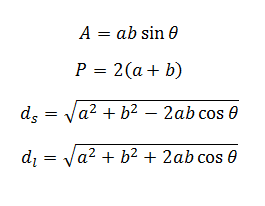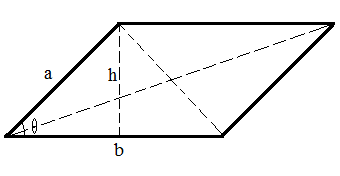# Parallelogram Calculator

This CalcTown calculator calculates the area, perimeter and diagonal lengths of a parallelogram

#### Result

m2
m
m
mWhere,

a, b = the sides of the parallelogram

θ = angle between the two sides

A = area of the parallelogram

P = perimeter of the parallelogram

ds = short diagonal length

dl = long diagonal length